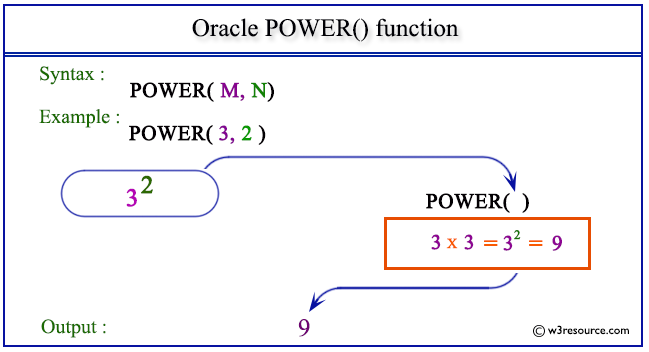# Oracle POWER() function

## Description

The Oracle POWER function is used to return the value of a number raised to the power of another number. Suppose base M and the exponent is N. The base M and the exponent N can be any numbers, but if M is negative, then N must be an integer.
The function takes any numeric or nonnumeric data type (can be implicitly converted to a numeric data type) as an argument.
If the argument is BINARY_FLOAT, then the function returns BINARY_DOUBLE. Otherwise, the function returns the same numeric data type as the argument

Syntax:

`POWER(M,N) `

Parameters:

Name Description
M A number which is the base of the exponentiation.
N A number which is the exponent of the exponentiation.

Pictorial Presentation of POWER() functionExample:

The statement below returns the value of 32, i.e. 9.

``````SELECT POWER(3, 2) FROM dual;
```
```

Here is the result.

```POWER(3,2)
----------
9
```

Example: POWER() function with negative value

The statement below returns the value of 4-2, i.e. 0.0625.

``````SELECT POWER(4,-2) from dual;
```
```

Here is the result.

```POWER(4,-2)
-----------
.0625
```

Previous: NANVL
Next: REMAINDER

﻿